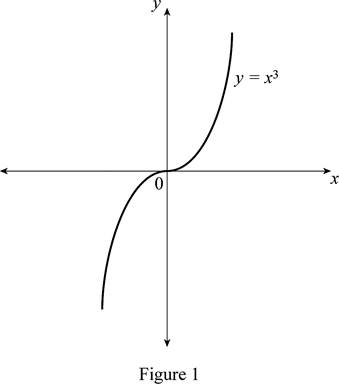# The function y = x 3 by hand without plotting any points and then graph of the function y = x 3 + 1 .### Single Variable Calculus: Concepts...

4th Edition
James Stewart
Publisher: Cengage Learning
ISBN: 9781337687805### Single Variable Calculus: Concepts...

4th Edition
James Stewart
Publisher: Cengage Learning
ISBN: 9781337687805

#### Solutions

Chapter 1.3, Problem 11E
To determine

## To sketch: The function y=x3 by hand without plotting any points and then graph of the function y=x3+1 .

Expert Solution

### Explanation of Solution

The standard graph of the function y=x3 is roughly drawn as shown below in Figure 1.Since the number 1 is added to y=x3 , shift the graph y=x3 by 1 unit upward. Thus, the graph of y=x3+1 is shown below in Figure 2.Thus, Figure 2 is obtained from Figure 1 by shifting 1 unit upward.

### Have a homework question?

Subscribe to bartleby learn! Ask subject matter experts 30 homework questions each month. Plus, you’ll have access to millions of step-by-step textbook answers!